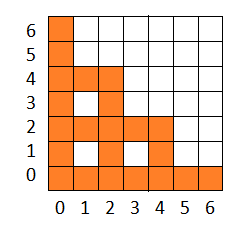시간 제한 메모리 제한 제출 정답 맞은 사람 정답 비율
1 초 512 MB 0 0 0 0.000%

## 문제

Young inventor has created a new jumping machine. In order to test it, he brought it to the testing polygon. The polygon is an infinite square grid.

Initially, the machine is located in the cell $(0, 0)$. The machine has $n$ springs, the $i$-th spring has the force of $l_i$ and allows the machine to jump $l_i$ cells up or $l_i$ cells to the right. Therefore this spring allows the machine to get from cell $(x, y)$ either to cell $(x + l_i, y)$, or to cell $(x, y + l_i)$. After jumping, the spring is thrown back and cannot be reused. The machine can use the springs in any order.

During the tests the cells, that the machine will fly over, will be stained with machine oil. In order not to clean the grid after himself, the inventor has decided to put a protective mat on each cell the machine could potentially fly over.

Now the inventor is wondering how many protective mats he needs to bring to the test with him.

## 입력

The first line of input contains $n$ --- the number of springs that the machine has ($1 \le n \le 100$). The second line contains $n$ integers $l_i$ --- the springs forces ($l_i \ge 1$; $1 \le l_1 + l_2 + \dots + l_n \le {10}^6$).

## 출력

Output a single integer: the number of mats that inventor needs to bring.

## 예제 입력 1

2
4 2


## 예제 출력 1

22


## 힌트

All the cells that can get dirty when the machine jumps in the example test are colored orange one the figure below.Cells that the machine can stain.